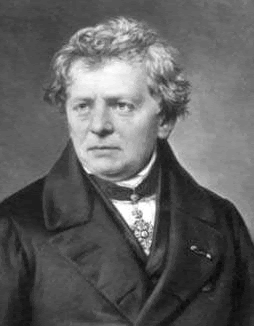### FCC Technician Exam Question Of The Day (T5D03)

Q) What formula is used to calculate resistance in a circuit?

A) Resistance (R) equals voltage (E) divided by current (I)

NJ2X Notes:
Ohm's Law was discovered by Georg Simon Ohm and is worth committing to memory:

E = I * R

or by manipulating the terms algebraically:

I = E / R

or

R = E / IGeorg Simon Ohm